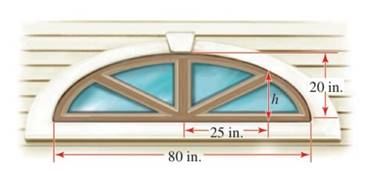Chapter 12.2, Problem 69E### Algebra and Trigonometry (MindTap ...

4th Edition
James Stewart + 2 others
ISBN: 9781305071742

#### Solutions

Chapter
Section### Algebra and Trigonometry (MindTap ...

4th Edition
James Stewart + 2 others
ISBN: 9781305071742
Textbook Problem

# Sunburst Window A “sunburst” window above a doorway is constructed in the shape of the top half of an ellipse, as shown in the figure. The window is 20 in. tall at its highest point and 80 in. wide at the bottom. Find the height of the window 25 in. from the center of the base.To determine

To Find:

The height of the window from the center of the base.

Explanation

Given:

The length of the major axis is 80 and the length of the semi minor axis is 20.

The height of window from the base is x=25.

Approach:

The equation of an ellipse is,

x2a2+y2b2=1… (1)

The length of the major axis is 2a.

The length of the minor axis is 2b.

Calculation:

Since,

2a=80a=40

And b=20 which is the height of the window.

From equation (1) the equation of the ellipse is given by,

x2402+y2202=1y2202=1

### Still sussing out bartleby?

Check out a sample textbook solution.

See a sample solution

#### The Solution to Your Study Problems

Bartleby provides explanations to thousands of textbook problems written by our experts, many with advanced degrees!

Get Started